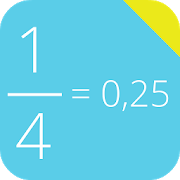#### Alphabetical

A | B | C | D | E | F | G | H | I | J | K | L | M | N | O | P | Q | R | S | T | U | V | W | X | Y | Z

# Decimal to FractionThis free mathematical calculator is able to convert decimal numbers into fractions. <br><br>Best math tool for school and college! If you are a student, it will help you to learn arithmetic and division!